Language :

Railway Group D & ALP Exam 2018 Important Questions of Reasoning Ability - Set 8Question 1Directions (1 to 2): In the following questions, select the related letters/word/number from the given alternatives. 2 : 7 : : 6 : ?Directions (1 to 2): In the following questions, select the related letters/word/number from the given alternatives. 2 : 7 : : 6 : ?Read Less
 A40B39C18D72
 Question 2ABCD : WXYZ : : EFGH : ?ABCD : WXYZ : : EFGH : ?Read Less
 ASTUVBSTOUCSTUEDTSUV
 Question 3A series is given in the following questions in which one term is omitted. Find the correct option from the given options which completes the series? n __ tns __ __ s __ nstn __ tnsA series is given in the following questions in which one term is omitted. Find the correct option from the given options which completes the series? n __ tns __ __ s __ nstn __ tnsRead Less
 AtstsnBstntsCsntnsDtnstn
 Question 4Find the missing number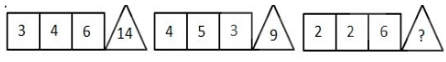Find the missing numberRead Less
 A6B5C8D4
 Question 5Two positions of a dice are shown below. When 3 is at the bottom, what number will be at the top?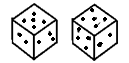Two positions of a dice are shown below. When 3 is at the bottom, what number will be at the top?Read Less
 A6B3C4D2
 Question 6In a symbolic language, A ÷ B means add A to B, A – B means multiply A by B, A x B means divide A by B and A + B means subtract B from A, then: 72 × 9 ÷ 9 – 6 + 2 = ?In a symbolic language, A ÷ B means add A to B, A – B means multiply A by B, A x B means divide A by B and A + B means subtract B from A, then: 72 × 9 ÷ 9 – 6 + 2 = ?Read Less
 A60B42C12D48
 Question 7Pointing towards a person in a photograph, Archana said, “that person is the only son of the father of the brother of my sister”. How is that person related to Archana? Pointing towards a person in a photograph, Archana said, “that person is the only son of the father of the brother of my sister”. How is that person related to Archana? Read Less
 AMaternal brotherBMotherCBrotherDData insufficient
 Question 8How many rectangles, that are not Hsquares, are there in the given diagram?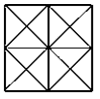How many rectangles, that are not Hsquares, are there in the given diagram?Read Less
 A10B14C12D8
 Question 9Which of the given answer figures will be the correct mirror reflection?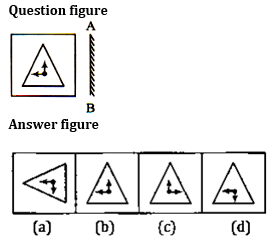Which of the given answer figures will be the correct mirror reflection?Read Less
 AaBbCcDd
 Question 10In the following question given below, a figure is given whose some part is missing. From the given answer figures, which option will complete the pattern?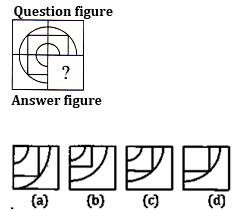In the following question given below, a figure is given whose some part is missing. From the given answer figures, which option will complete the pattern?Read Less
 AaBbCcDd

## Railways Exam - ONLINE TEST

IBT Institute offer you the most trusted and highly recommended Test Series for Railways Exam. 8 out of every 10 candidates selected in Railways Exam last year opted for IBT Online Test Series.

## Railways Exam - CLASS ROOM COURSE

Keeping in mind the Railways Exam, IBT has launched Special batches for Railways Exam to guide you through your preparations. Seats filling fast. Don’t miss out!!

SSC CGLE TIER-19 Books

As per Latest Exam PatternTo Watch Latest Videosby Expert TrainersCLICK HERE TO SUBSCRIBE